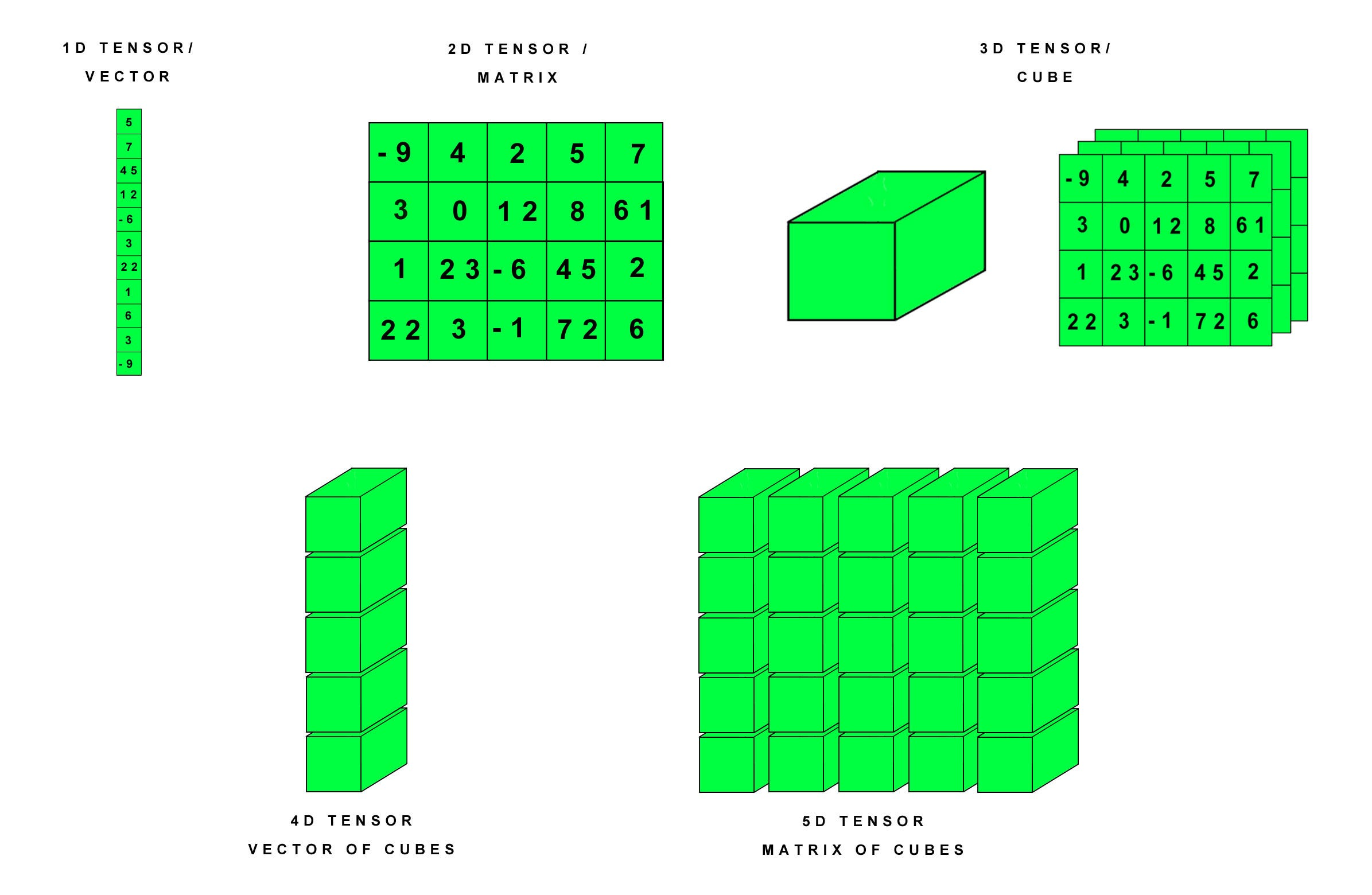1591344961

Getting Started with PyTorch
PyTorch is a relatively new and robust deep learning framework, having a dynamic computation graph and supports flexibility. It was designed primarily by Soumith Chinatala, Facebook AI Research.

#tensor #pytorch #machine-learning #programming

## Buddha Community1591344961

Getting Started with PyTorch
PyTorch is a relatively new and robust deep learning framework, having a dynamic computation graph and supports flexibility. It was designed primarily by Soumith Chinatala, Facebook AI Research.

#tensor #pytorch #machine-learning #programming1595580780

## Tensor operations in PyTorch

PyTorch is a scientific package based on Python, which is used to perform advanced operations using a special datatype known as Tensor. A Tensor is a number, vector, matrix, or multi-dimensional array with regular shape and numbers of the same datatype. PyTorch is an alternative to the NumPy package, which can additionally be used with the power of GPUs. It is also used as a framework for conducting research in deep learning.Understanding tensors

The 5 operations are:

• expand()
• permute()
• tolist()
• narrow()
• where()

# 1. expand()

The existing tensor is expanded to new dimensions along the dimension with value 1. The tensor can be expanded along any one dimension or multiple dimensions at once. If you do not want to expand the tensor along a particular dimension, you can set its parametric value to -1.

Note: only singleton dimension can be expanded

In this example, the tensor has original dimensions as [1,2,3]. It is expanded to dimensions [2,2,3].

This function can be used to expand existing tensors along singleton dimensions. It only returns a new view and does not allocate new memory. Therefore, it can be used to study how the tensor behaves at larger dimensions.

# 2. permute()

This function returns a view of the tensor with the order of dimensions of original tensor changed according to our choice. For example, if the original dimensions are [1,2,3], we can change it to [3,2,1]. The function takes the required order of dimensions as its parameters.

#deep-learning #tensor #operations #jovian #pytorch #deep learning1619565060

## Ternary operator in Python?

1. Ternary Operator in Python

What is a ternary operator: The ternary operator is a conditional expression that means this is a comparison operator and results come on a true or false condition and it is the shortest way to writing an if-else statement. It is a condition in a single line replacing the multiline if-else code.

syntax : condition ? value_if_true : value_if_false

condition: A boolean expression evaluates true or false

value_if_true: a value to be assigned if the expression is evaluated to true.

value_if_false: A value to be assigned if the expression is evaluated to false.

How to use ternary operator in python here are some examples of Python ternary operator if-else.

Brief description of examples we have to take two variables a and b. The value of a is 10 and b is 20. find the minimum number using a ternary operator with one line of code. ( **min = a if a < b else b ) **. if a less than b then print a otherwise print b and second examples are the same as first and the third example is check number is even or odd.

#python #python ternary operator #ternary operator #ternary operator in if-else #ternary operator in python #ternary operator with dict #ternary operator with lambda

1607510226

## Best Web App Ideas To Make Money In 2021 - Application Startup Guide

Some Popular Web App Ideas for 2021

Are you looking for best web application business ideas that make money in 2021?

There are lots of simple web app ideas but all those web application business ideas do not make money.